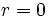# Differentiation rule for power functions

## Statement

We have the following differentiation rule:$\! \frac{d}{dx}(x^r) = rx^{r-1}$

where$r$ is a constant. Some notes on the validity:

Case on$r$ Values of real$x$ for which this makes sense$r = 0$ all nonzero$x$. Also makes sense at$x = 0$ if we interpret the left side as 1 (constant equal to the list at 0) and the right side as 0.$r$ a rational number with odd denominator and greater than or equal to 1 All$x$$r$ a real number greater than 1 that is not rational with odd denominator All$x > 0$. One-sided derivative makes sense at 0.$r$ a rational number with odd denominator and between 0 and 1 All$x \ne 0$. At 0, we have a vertical tangent or vertical cusp depending on the numerator of the rational function.$r$ a real number between 0 and 1 that is not rational with odd denominator All$x > 0$. One-sided vertical tangent at 0.$r$ a rational number with odd denominator and less than 0 All$x \ne 0$. At 0, we have a vertical asymptote$r$ a real number less than 0 that is not rational with odd denominator All$x > 0$. One-sided vertical asymptote at 0.